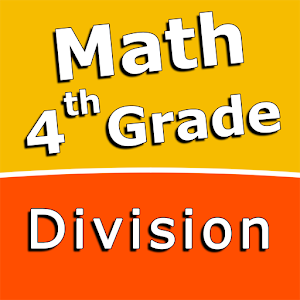Türkçe

Her gün ücretsiz lisanslı Android uygulamaları ve oyunlar sunuyoruz.\$2.99
SÜRESI DOLMUŞ

# Android Giveaway of the Day - Fourth grade Math skills - Division

Practice and improve your math skills!
\$2.99 SÜRESI DOLMUŞ
Kullanıcı puanı: 0

Bu programın süresi doldu. Fourth grade Math skills - Division artık firmadan temin edilebilir.

Intuitive interface powered by handwriting input and a choice of three fun and engaging mini games in addition to a regular math trainer mode make our app stand out from the crowd of generic math learning apps.

With Fourth grade Math skills - Division you can practice and improve the following math skills:
- Division facts to 12
- Divide two-digit numbers by one-digit numbers
- Divide three-digit numbers by one-digit numbers
- Divide three-digit numbers by two-digit numbers
- Divide four-digit numbers by one-digit numbers
- Divide four-digit numbers by two-digit numbers
- Divide numbers ending in zeroes by numbers up to 12

Choose between four different modes:
- Math Trainer
- Math Shot mini-game
- Math Gravity mini-game
- Math Trick Shot mini-game

Educational

## Fourth grade Math skills - Division üzerine yorumlar

Thank you for voting!